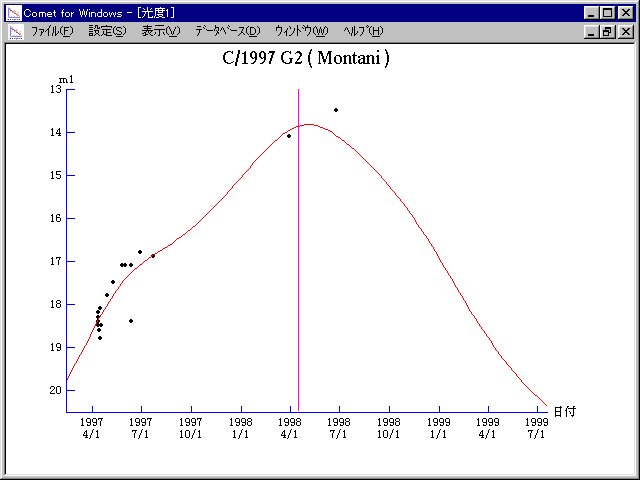# \$B%b%s%?!<%KWB@1(B

C/1997 G2 ( Montani )###\$B%W%m%U%#!<%k(B

 \$BH/8+F|(B 1997\$BG/(B4\$B7n(B12\$BF|(B \$BH/8+8wEY(B 18.4\$BEy(B \$BH/8+ Joe Montani

###\$B50F;MWAG(B

```   The following improved orbital elements by Kenji Muraoka, are
from 171 observations 1997 Apr. 12 to 1998 Oct. 18, perturbations
by 9 Planets, Moon and 5 minor planets were taken into account.
The mean residual is +/- 0.75 arc seconds.

Epoch  =  1998 Apr. 17.0  TT       JDT = 2450920.5
T  =  1998 Apr. 16.34313       +/- 0.00106 (m.e.) TT
Peri. =  239.85527                +/- 0.00018
Node  =   55.80386                +/- 0.00007   (2000.0)
Incl. =   69.83881                +/- 0.00015
q  =    3.0847713              +/- 0.0000061 AU
e  =    0.9942334              +/- 0.0000099
1/a  =   +0.0018694              +/- 0.0000032 1/AU
( P  =  12372 years )
orig. 1/a  =   +0.002513   1/AU
fut.  1/a  =   +0.002671   1/AU
```

###\$B@1?^(B###\$B8wEYJQ2=(B

```        m1 = 0.5 + 5 log\$B&\$(B + 22.5 log r
```##### \$B50F;MWAG\$OB<2,7r<#;a\$N7W;;\$K\$h\$k\$b\$N\$G\$9!#(B \$B@1?^\$O(B StellaNavigator Ver.2.0 for Windows (\$B%"%9%H%m%"!<%D(B \$BJTCx(B / \$B%"%9%-!<=PHG6I4)(B) \$B\$G:n@.\$7\$?\$b\$N\$G\$9!#(B \$B8wEY%0%i%U\$O(BComet for Windows\$B\$G:n@.\$7\$?\$b\$N\$G\$9!#(B